• Call Now

1800-102-2727•

# Convex lens, terminology, Image formation, Lens formula, Practice problems, FAQs

Have you burned paper with the help of magnifying lens in your childhood? Have you thought about how it is possible? When a light ray from the Sun comes on a converging lens it is nearly parallel. Hence the energy associated with it is also distributed over the area. After the refraction from the converging lens the rays are concentrated on a point, along with that energy also gets concentrated on a single point. Now if this point falls on a paper, it will start burning. Let's understand the convex lens in detail!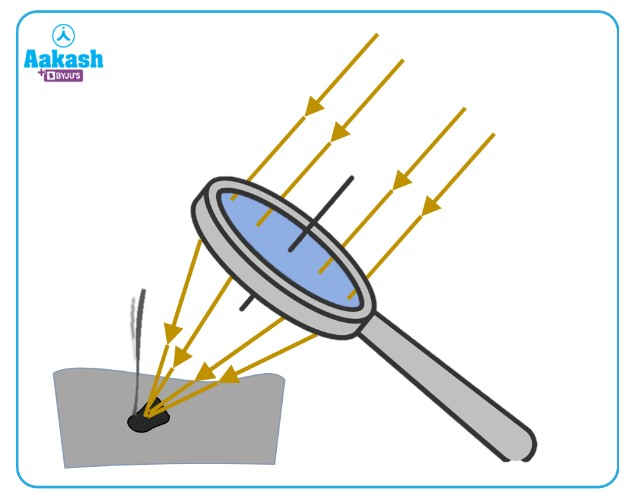Table of content

• Introduction
• Important Terminologies Used for Convex Lenses
• Image Formation in Convex Lens
• Lens formula for convex lens
• Practice problems
• FAQs

## Introduction

A convex lens is a transmissive optical device that focuses a light beam by means of refraction.

A convex lens is made up of two convex surfaces. These two concave surfaces are the part of two spheres as shown in the diagram.The radius of both the spheres can be the same or can be different. A convex lens is thin at the edges and is thick in the middle. Also, these lenses have a property that they can converge all the rays falling on it. Therefore, these types of lenses are also known as converging lenses.The types of converging lenses are shown in the figure below.Now let's see the terms related to convex lenses.

## Important Terminologies Used for Convex Lenses

1. Center of curvature (C): Convex lenses are a combination of two spherical surfaces as shown in the figure. These spherical surfaces are parts of two different spheres. Center of these shapes is known as the center of curvature. The center of curvature of the first surface is C1, and the center of curvature of the second surface is C2.For the lenses, having one surface as plane, and the other surface as spherical. The center of the plane surface is at infinity. For the spherical part, the center is at C as shown in the figure2. Radius of curvature: The distance between the center of the lens and the center of curvature is known as the radius of curvature.

3. Principal axis: The line joining the center of curvatures of two bounding surfaces is known as the principal axis.

4. Optical center: An optical center is a point for a given lens through which any ray passes without any deviation. For a biconcave or a biconvex lens, the optical center of the lens is located at the geometrical center of the lens.Note:

The optical center can lie inside, on the surface, or outside the lens, depending upon the values of R1 and R2.

5. Focus: Lens have two focuses because the light can strike the lens from both the sides. They are known as first principal focus and second principal focus.

First principal focus:

For a convex lens, it is the point on the principal axis where the object is kept; the rays from that object become parallel to the principal axis after passing through the lens (i.e., the image forms at infinity).## Second principal focus

For a convex lens, it is the point on the principal axis where the rays parallel to the principal axis converge after passing through the lens.Note: The second principal focus is the main focus. In a question, if they mention focus, we have to take that as the second principal focus.

6. Focal plane: The rays parallel to each other and not parallel to the principal axis converge at a point after passing through the lens that lies on the plane containing the focus of the lens. This plane is known as the focal plane.## Image Formation in Convex Lens

Position of the image from a convex lens depends upon the position of the object. Here are some cases that are discussed for image formation.

1. When an object is at infinity

If the object is at infinity from the lens, the rays coming from the distant object are parallel to the principal axis. Therefore, after passing through the lens, they converge at the focus.The characteristics of the image formed are as follows:

1. The image is formed at F.
2. The image is real and inverted.
3. The image formed is highly diminished.

2. When an object is beyond 2F

It takes two rays to trace the image from the object. The first ray is parallel to the principal axis that passes through the focus after passing through the lens. The second ray passes through the optical center of the lens. Both the rays meet each other at some point where the image is formed.The characteristics of the image formed are as follows:

1. The image is formed between F and 2F.
2. The image is real and inverted.
3. The image formed is diminished.

3. When an object is at 2F

Again we have to take two rays.The first ray is parallel to the principal axis that passes through the focus after passing through the lens. The second ray passes through the optical center of the lens. Both the rays meet each other at some point where the image is formed.The characteristics of the image formed are as follows:

1. The image is formed at 2F.
2. The image is real and inverted.
3. The image formed is of the same size.

4. When an object is between 2F and F

Again we have to take two rays. The first ray is parallel to the principal axis that passes through the focus after passing through the lens. The second ray passes through the optical center of the lens. Both the rays meet each other where the image is formed.The characteristics of the image formed are as follows:

1. The image is formed beyond 2F.
2. The image is real and inverted.
3. The image formed is enlarged.

5. When an object is at F

Again we have to take two rays. The first ray is parallel to the principal axis that passes through the focus after passing through the lens. The second ray passes through the optical center of the lens. Both the rays meet each other at a very distant point, i.e., infinity.The characteristics of the image formed are as follows:

1. The image is formed at infinity.
2. The image formed is real and inverted.
3. The image formed is highly enlarged.

6. When an object is between F and the optical center

Again we have to take two rays. The first ray is parallel to the principal axis that passes through the focus after passing through the lens. The second ray passes through the optical center of the lens. In this case, the rays do not meet at any point in real, but when they retrace back, they appear to meet behind the object.The characteristics of the image formed are as follows:

1.The image is formed behind the object.
2. The image formed is virtual and erect.
3. The image formed is enlarged.

Now let's see the formula for calculating position of image.

## Lens formula for convex lens

The relation between the focus, object distance and image distance is known as lens formula, and is given as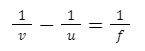Where,

v : x-coordinate of the image

u : x-coordinate of the object

f : focal length

Sign convention:  The direction of the incident ray is taken as positive and optical center as origin. For convex lens focal length is positive and for concave lens it is negative.

## Practice problems

Q 1.The figure shows a point object and a converging lens. Find the position of the final image formed.

Answer: The ray tracing from the point object is shown in the figure.

Therefore, the positive x-axis is towards the right side. Hence, u = - 15 cm

Since the lens is converging, f = + 10 cm.

By applying the lens formula, we get,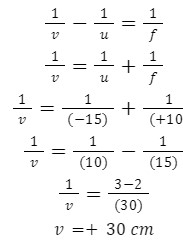Therefore, the image forms on the positive side 30 cm away from the optical center.

Q 2. An object is placed at a distance 12 cm from the convex lens of focal length 8 cm. Find the position of the image.

For convex lens focal length will be positive, hence f=8 cm

u=-12 cm

Using lens formula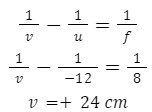(+ve sign shows that image is formed behind the lens)

Hence the image will be real and inverted.

Q 3. If the virtual image is needed to be formed by a convex lens of focal length 10 cm at a distance 10 cm in front of the mirror. Where should the object be placed?

Given f=10 cm

v=-10 cm

Using lens formula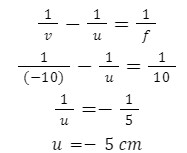Object will also be placed in front of mirror at a distance of 5 cm.

Q 4. For an object at 20 cm distance in front of a convex lens, the image formed at 20 cm behind the lens. The focal length of a convex lens is.

Given u=-20 cm

v=+20 cm

Using lens formula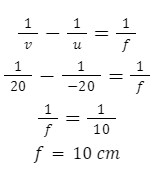## FAQs

Q 1. How can someone identify a convex lens physically?
Answer: Convex lens is thick at the center and thin at the corner.

Q 2. Which type of lens does the human eye have?
Human eye has a Convex lens

Q 3. Why a convex lens is used in a simple camera?
Convex lenses are used in cameras so that the real image that forms can be taken on screen.

Q 4. What are the various uses of convex lenses?
Convex lens is used in magnifying glasses, eye glasses, cameras, microscopes etc.Talk to our expert
Resend OTP Timer =
By submitting up, I agree to receive all the Whatsapp communication on my registered number and Aakash terms and conditions and privacy policy